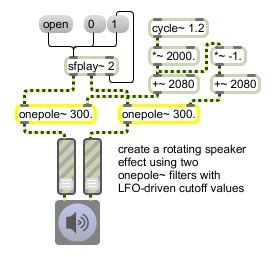# onepole~

Single-pole lowpass filter

## Description

The onepole~ implements the simple filter equation

output = previous input + cf * (input - previous input)

where cf represents the cutoff frequency of the filter expressed in radians. The values for cf lie in the range -1.0-0. This produces a single-pole lowpass filter with a 6dB/octave attenuation, which can be useful to gently roll off harsh high end (e.g., the digital artifacts of downsampling). onepole~ is equivalent to a biquad~ object with the coefficients,

[a0 = 1 + cf, a1 = 0, a2 = 0, b1 = cf, b2 = 0]

If you substitute these values into the biquad~ equation, you are left with the onepole~ object's algorithm. However, onepole~ will execute much faster, since biquad~ will still compute the unused portion of its equation.

## Arguments

Name Type Opt Description
center-frequency (Hz) float opt Sets the center frequency for the filter, as described above.
Hz/linear/radians symbol opt Using the symbols Hz, linear, or radians for an optional second argument sets the frequency input mode. The default mode is Hz (which is the same as providing no mode argument). Using the linear argument sets the frequency input mode to linear (0 - 1). Using the radians argument sets the frequency input mode to radians (0 - 1).

## Messages

 int center-frequency (Hz) [int] Performs the same function as float. float center-frequency (Hz or 0 through 1 specified by object-argument) [float] In right inlet: Sets the frequency for the filter (if no signal is connected). By default, frequency is expressed in Hz, where the allowable range is from 0 to one fourth of the current sampling rate. For convenience, onepole~ has two additional input modes that use the more conventional input range, 0 - 1 (see the linear and radians messages). Hz In either inlet: Sets the frequency input mode to Hz (the default). clear In either inlet: Clears the internal state of onepole~. Since onepole~ does not have the inherent instability of other filter types, this should never be necessary. linear In either inlet: Sets the frequency input mode to linear (0 - 1). Linear mode is simply a scaled version of the standard Hz mode, except that values in the 0-1 range traverses the full frequency range. radians In either inlet: Sets the frequency input mode to radians (0 - 1). Radians mode lets you set the center frequency (cf) of the equation directly, while the input has the same range (0-1), the output has a curved frequency response that is closer to the exponential pitch scale of the human ear. signal In left inlet: Signal to be filtered. In right inlet: A signal can be used to set the frequency for the filter, with the same effect as a float. If a signal is connected to this inlet, its value is sampled once every signal vector.

## Output

signal: The filtered signal.

## Examplesonepole~ provides efficient filtering for a simple sample player